# Set Thickness of Plots in R

R programming is considered one of the most useful and widely-used programming languages for data and statistical analysis. One essential feature for carrying out such analysis is the visualization of data using beautiful graphs and figures.

In R language, the `matplot()`, `plot()`, `ggplot()` are some of the most commonly used functions for plotting different graphs.

The width of axes and lines in such graphs is usually dependent on the type of device. We can alter the thickness of plot lines using the `lwd` parameter in different functions.

In this tutorial, we will introduce how to set the thickness of the plot lines, the axes, and also the borders of the graph.

## Set the Thickness of Plot Lines

We can use the `lwd` parameter to set the thickness of plot lines. For example, first, we will plot a simple line graph using the `plot()` function. The `sample()` function here creates a random distribution of 100 elements:

``````plot(sample(100), type = 'l')
``````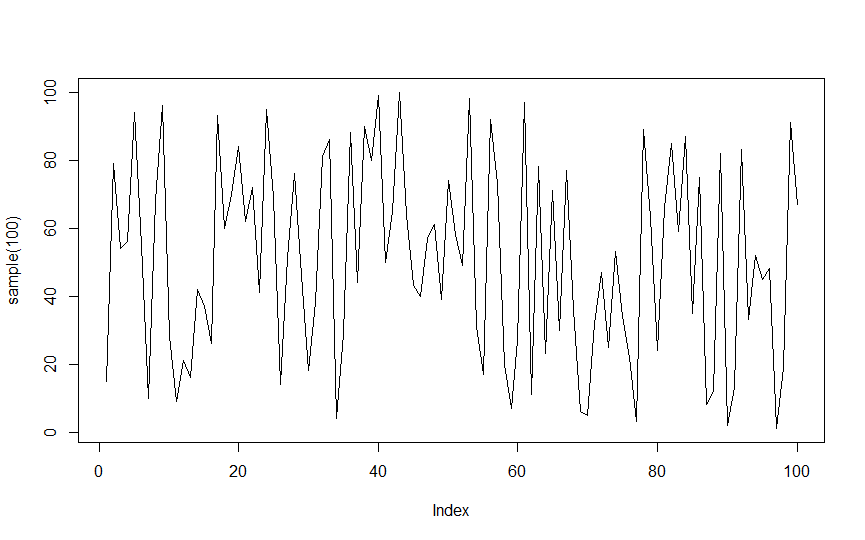Now to change the thickness of the plot line, we will insert the `lwd` parameter to the `plot()` function and set it to the desired value:

``````plot(sample(100), type = 'l',lwd = 4)
``````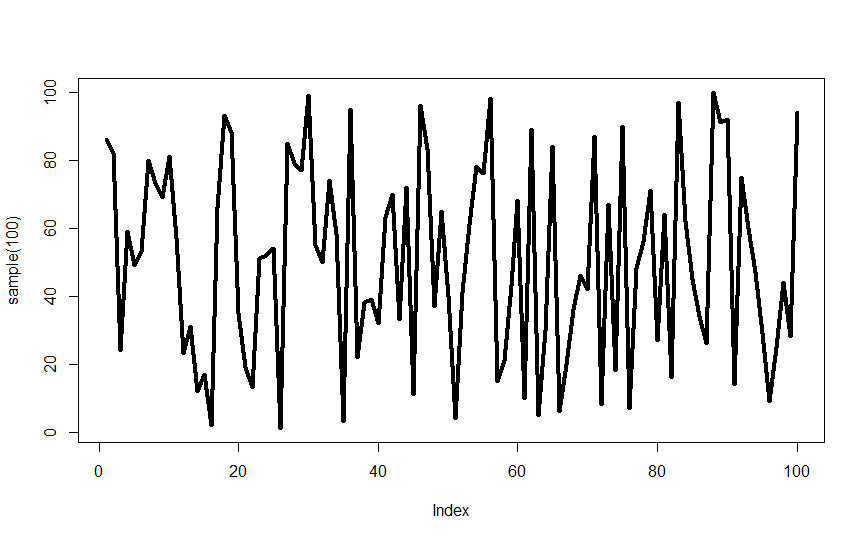Notice the increase in the thickness of the plot lines. Similarly, we can also set the thickness of the axes and borders.

## Altering the Thickness of Axes

First, we will start with a simple scatter plot of a random distribution of 100 elements created using the `sample()` function. We will use the `plot()` function to plot this distribution.

``````plot(sample(100))
``````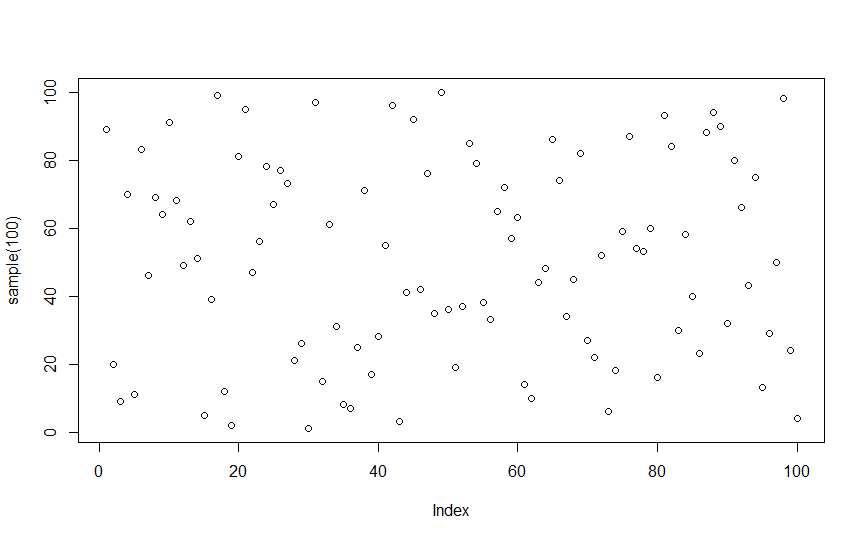Now we will use the function `axis()`. This allows us to impose an axis to the current plot at a specified position or thickness, with even more customizations available. To set the axis’s thickness, we will change the `lwd` parameter and set it to our desired thickness.

``````plot(sample(100))
axis(side = 1, lwd = 3)
axis(side = 2, lwd = 3)
``````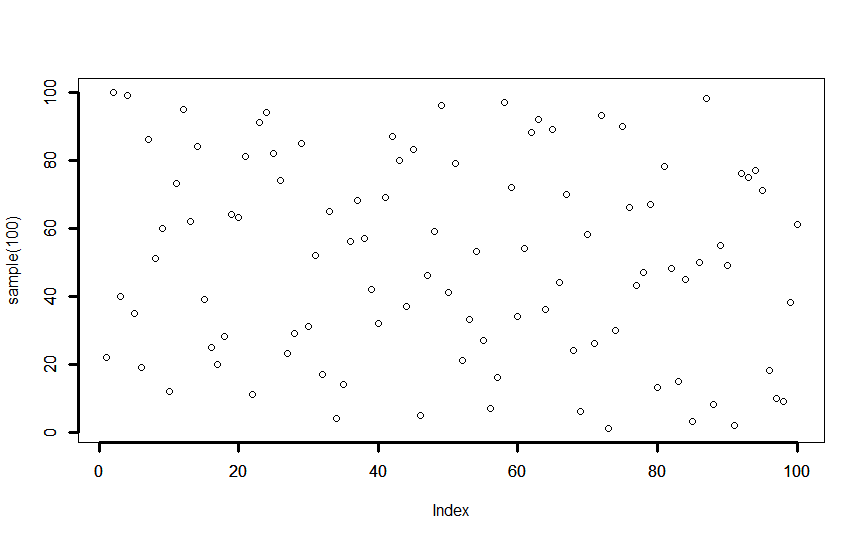## Altering the Thickness of the Borders

We can add the `box()` function to add a box around the graph with some desired thickness.

For example, we can add a box to a BoxPlot graph (we have also increased the thickness of the boxplot using boxlwd) as shown below:

``````boxplot(sample(100), horizontal = TRUE, notch = TRUE, boxlwd = 4)
box(lwd=2)
``````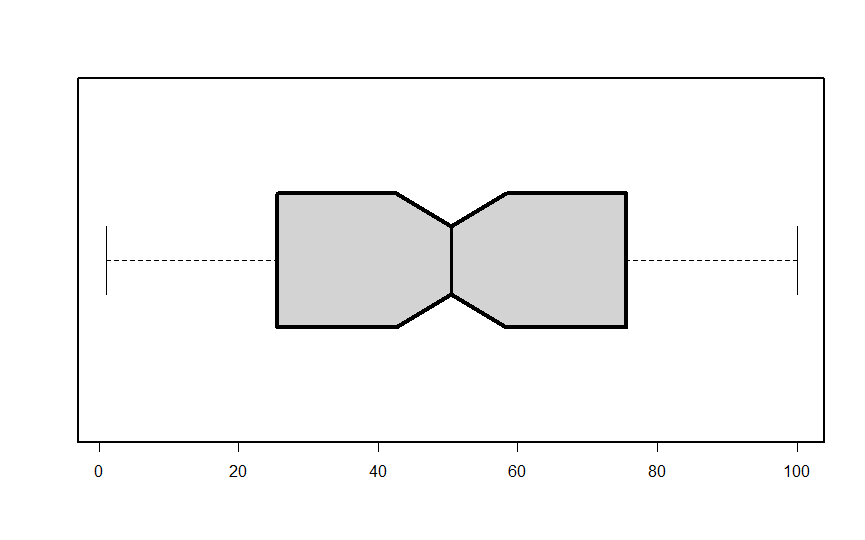## Related Article - R Plot

• Create Stacked Histogram in R